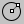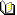Circle

Creates a circle.

Steps:

• Follow the prompts for the selected option. If no option is specified, the default option is used.

Options

Creates a circle from a center location and a radius.

1. Pick the center.

2. Pick a radius or diameter.

Deformable

Vertical

Draws a circle perpendicular to the construction plane.

1. Pick the center.

2. Pick a radius or diameter.

2Point

Draws a circle from the two ends of its diameter.

1. Pick the start of the diameter.

2. Pick the end of the diameter.

3Point

Draws a circle through three points on the circumference.

1. Pick the first point.

2. Pick the second point.

3. Pick the third point.

Tangent

Draws a circle tangent to curves.

1. Pick the first tangent location on the first curve.

2. Pick the second tangent curve, or type a radius.

3. Pick the third tangent curve, or press Enter

to draw the circle from the first and second tangent locations.

Options

Note: The options are the same as the command.

Pick any point. This point does not have to be a tangent point on a curve.

Forces the circle or arc to go through the first picked point on the curve instead of allowing the point to slide along the curve.

The circle is restricted to the specified radius. If a tangent point exists on the second curve that meets the radius requirement, the tangent constraint will appear at that point as you drag the circle.

AroundCurve

Draws a circle perpendicular to a curve.

1. Select

the curve.

2. Pick
the center on a curve.

.

FitPoints

Draws a circle from fit to point objects.

• Select point objects.

• A minimum of three points is required.Circle > Circle: Center, Radius Main1 > Circle: Center, RadiusCurve > Circle > Center, RadiusRelated topics…